# Brauer second main theorem

Letbe an element ofwhose order is a power of. The-section ofassociated tois the set of all elements ofwhose-part is conjugate to. Brauer second main theorem relates the values of irreducible characters ofon the-section associated toto values of characters in certain blocks of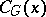.
Suppose thatis an irreducible character of(cf. also Character of a group), afforded by the-free right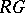-module, and belonging to the block(cf. also Defect group of a block). Letbe a-element of, and let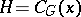. For all-subgroupsof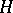,; hence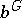is defined for all blocks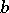of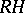. One can organize the block decomposition ofas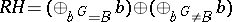. Letbe the projection ofon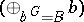, and letbe the projection ofon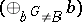. The restriction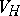oftocan be decomposed as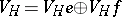. Ifis the character of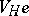andis the character of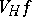, then of course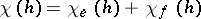for all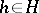. Brauer's second main theorem states that for all elementsof order prime to,. Thus, the values ofon the-section associated toare determined in the blocks ofsent toby the Brauer correspondence (cf. also Brauer first main theorem).